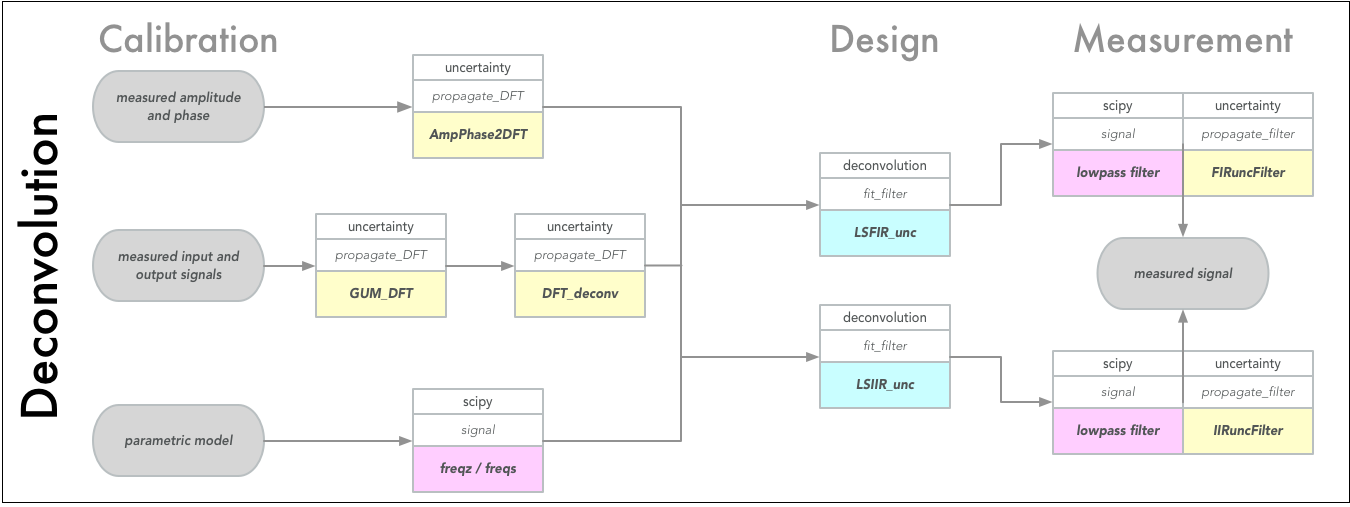# Examples¶

On the project website in the examples subfolder and the separate PyDynamic_tutorials repository you can find various examples illustrating the application of PyDynamic. Here we provide only a quick starter.

## Quick Examples¶

Uncertainty propagation for the application of an FIR filter with coefficients b with which an uncertainty ub is associated. The filter input signal is x with known noise standard deviation sigma. The filter output signal is y with associated uncertainty uy.

```from PyDynamic.uncertainty.propagate_filter import FIRuncFilter
y, uy = FIRuncFilter(x, sigma, b, ub)
```

Uncertainty propagation through the application of the discrete Fourier transform (DFT). The time domain signal is x with associated squared uncertainty ux. The result of the DFT is the vector X of real and imaginary parts of the DFT applied to x and the associated uncertainty UX.

```from PyDynamic.uncertainty.propagate_DFT import GUM_DFT
X, UX = GUM_DFT(x, ux)
```

Sequential application of the Monte Carlo method for uncertainty propagation for the case of filtering a time domain signal x with an IIR filter b,a with uncertainty associated with the filter coefficients Uab and signal noise standard deviation sigma. The filter output is the signal y and the Monte Carlo method calculates point-wise uncertainties uy and coverage intervals Py corresponding to the specified percentiles.

```from PyDynamic.uncertainty.propagate_MonteCarlo import SMC
y, uy, Py = SMC(x, sigma, b, a, Uab, runs=1000, Perc=[0.025,0.975])
```## Detailed examples¶

More comprehensive examples you can find in provided Jupyter notebooks, which require additional dependencies to be installed. This can be achieved by appending `[examples]` to PyDynamic in the install command, e.g.

```pip install PyDynamic[examples]
```

Afterwards you can browser through the following list: